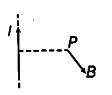# A long straight wire carries a current of 35 A

A long straight wire carries a current of 35 A . What is the magnitude of the field B at a point 20 cm away from the wire?

Here, we have to find the magnetic field at point P.
I = 35 A and r = 20 cm= 0.2 m The wire is long and it is considered as an infinite length wire. The magnetic field
B = { µ }_{ 0 } / 4 \pi. 2I/ r
= {{10}^{-7}} x 2 x 35 / 0.2 = 3.5 x {{10}^{-5}} T
The direction of magnetic field is given by right hand thumb rule. If current is upwards, then magnetic field at P is perpendicularly inwards to the plane of paper.